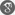##### Cite as:
Lakshmi Narayan Mishra, H. M. Srivastava, Mausumi Sen, Existence Results for Some Nonlinear Functional-Integral Equations in Banach Algebra with Applications, Int. J. Anal. Appl., 11 (1) (2016), 1-10.

#### Abstract

In the present manuscript, we prove some results concerning the existence of solutions for some nonlinear functional-integral equations which contains various integral and functional equations that considered in nonlinear analysis and its applications. By utilizing the techniques of noncompactness measures, we operate the fixed point theorems such as Darbo's theorem in Banach algebra concerning the estimate on the solutions. The results obtained in this paper extend and improve essentially some known results in the recent literature. We also provide an example of nonlinear functional-integral equation to show the ability of our main result.

#### References

1. R.P. Agarwal, N. Hussain, M.A. Taoudi, Fixed point theorems in ordered Banach spaces and applications to nonlinear integral equations, Abstr. Appl. Anal. 2012 (2012), Article ID 245872.2. R.P. Agarwal, D. O’Regan, P.J.Y. Wong, Positive Solutions of Differential, Difference and Integral Equations, Kluwer Academic, Dordrecht, 1999.3. G. Anichini, G. Conti, Existence of solutions of some quadratic integral equations, Opuscula Math. 28 (4) (2008), 433-440.4. J. Bana´s, A. Chlebowicz, On existence of integrable solutions of a functional integral equation under Carathéodory conditions, Nonlinear Anal. 70 (9) (2009), 3172-3179.5. J. Bana´s, K. Goebel, Measures of Noncompactness in Banach Spaces, Lecture Notes in Pure and Applied Mathematics, vol. 60, Marcel Dekker, New York, 1980.6. J. Bana´s, M. Lecko, Fixed points of the product of operators in Banach algebra, Panamer. Math. J. 12 (2) (2002), 101-109.7. J. Bana´s, M. Mursaleen, Sequence Spaces and Measures of Noncompactness with Applications to Differential and Integral Equations, Springer, New York, 2014.8. J. Bana´s, B. Rzepka, An application of a measure of noncompactness in the study of asymptotic stability, Appl. Math. Lett. 16 (1) (2003), 1-6.9. J. Bana´s, B. Rzepka, On existence and asymptotic stability of solutions of a nonlinear integral equation, J. Math. Anal. Appl. 284 (1) (2003), 165-173.10. J. Bana´s, B. Rzepka, On local attractivity and asymptotic stability of solutions of a quadratic Volterra integral equation, Appl. Math. Comput. 213 (1) (2009), 102-111.11. J. Bana´s, K. Sadarangani, Solutions of some functional-integral equations in Banach algebra, Math. Comput. Modelling 38 (2003), 245-250.12. V.C. Boffi, G. Spiga, An equation of hammerstein type arising in particle transport theory, J. Math. Phys. 24 (6) (1983), 1625-1629.13. T.A. Burton, B. Zhang, Fixed point and stability of an integral equation: nonuniqueness, Appl. Math. Lett. 17 (7) (2004), 839-846.14. S. Chandrasekhar, Radiative Transfer, Oxford Univ Press, London, 1950.15. C. Corduneanu, Integral Equations and Applications, Cambridge University Press, New York, 1990.16. G. Darbo, Punti uniti in trasformazioni a codominio non compatto, Rend. Sem. Mat. Univ. Padova 24 (1955), 84-92.17. M.A. Darwish, K. Sadarangani, Nondecreasing solutions of a quadratic Abel equation with supremum in the kernel, Appl. Math. Comput. 219 (14) (2013), 7830-7836.18. Deepmala, H.K. Pathak, A study on some problems on existence of solutions for nonlinear functional-integral equations, Acta Math. Sci. 33 (5) (2013), 1305-1313.19. K. Deimling, Nonlinear Functional Analysis, Springer-Verlag, New York, 1985.20. B. C. Dhage, On α-condensing mappings in Banach algebras, Math. Student 63 (1994), 146-152.21. D. Guo, V. Lakshmikantham, X.Z. Liu, Nonlinear Integral Equations in Abstract Spaces, Kluwer, Dordrecht, 1996.22. S. Hu, M. Khavani, W. Zhuang, Integral equations arising in the kinetic theory of gases, Appl. Anal. 34 (1989), 261-266.23. X.L. Hu, J.R. Yan, The global attractivity and asymptotic stability of solution of a nonlinear integral equation, J. Math. Anal. Appl. 321 (1) (2006), 147-156.24. C.T. Kelly, Approximation of solutions of some quadratic integral equations in transport theory, J. Integral Equations 4 (3) (1982), 221-237.25. A.A. Kilbas, H.M. Srivastava, J.J. Trujillo, Theory and Applications of Fractional Differential Equations, North-Holland Mathematical Studies, vol. 204, Elsevier (North-Holland) Science Publishers, Amsterdam, London and New York, 2006.26. Z. Liu, S.M. Kang, Existence and asymptotic stability of solutions to a functional-integral equation, Taiwanese J. Math. 11 (1) (2007), 187-196.27. Z. Liu, S.M. Kang, Existence of monotone solutions for a nonlinear quadratic integral equation of Volterra type, Rocky Mountain J. Math. 37 (6) (2007), 1971-1980.28. K. Maleknejad, R. Mollapourasl, K. Nouri, Study on existence of solutions for some nonlinear functional integral equations, Nonlinear Anal. 69 (8) (2008), 2582-2588.29. K. Maleknejad, K. Nouri, R. Mollapourasl, Existence of solutions for some nonlinear integral equations, Commun. Nonlinear Sci. Numer. Simul. 14 (6) (2009), 2559-2564.30. K. Maleknejad, K. Nouri, R. Mollapourasl, Investigation on the existence of solutions for some nonlinear-functional integral equations, Nonlinear Anal. 71 (12) (2009), e1575-e1578.31. L.N. Mishra, R.P. Agarwal, M. Sen, Solvability and asymptotic behavior for some nonlinear quadratic integral equation involving Erdélyi-Kober fractional integrals on the unbounded interval, Progr. Fract. Differ. Appl. In press.32. L.N. Mishra, M. Sen, On the concept of existence and local attractivity of solutions for some quadratic Volterra integral equation of fractional order, Appl. Math. Comput. 285 (2016) 174-183.33. L.N. Mishra, M. Sen, R.N. Mohapatra, On existence theorems for some generalized nonlinear functional-integral equations with applications, Filomat, in press.34. D. O’Regan, Existence results for nonlinear integral equations, J. Math. Anal. Appl. 192 (3) (1995), 705-726.35. D. O’Regan, M. Meehan, Existence Theory for Nonlinear Integral and Integrodifferential Equations, Kluwer, Dordrecht, 1998.36. H.K. Pathak, Deepmala, Remarks on some fixed point theorems of Dhage, Appl. Math. Lett. 25 (11) (2012), 1969-1975.37. P.P. Zabrejko, A.I. Koshelev, M.A. Krasnosel’skii, S.G. Mikhlin, L.S. Rakovshchik, V.J. Stetsenko, Integral Equations, Noordhoff, Leyden, 1975.